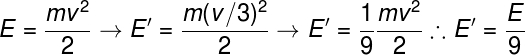Mechanics

# Kinetic energy

Kinetic energy is the form of energy related to moving bodies. Kinetic energy is a scalar physical quantity whose unit of measurement, according to SI units , is the joule . Kinetic energy is directly proportional to the square of the body ‘s velocity .

## Definition of kinetic energy

“Kinetic energy is the ability of a moving body to do work.”

Kinetic energy is the ability of a moving body to do work , changing the state of motion of bodies around it or deforming them . The greater the speed and mass of the body, the greater its ability to do work when in motion. In an analogous way, we can think that a body that presents a great kinetic energy needs a great amount of energy to stop its motion.

Here is the formula used to calculate kinetic energy: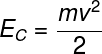C – kinetic energy (J)

m – body mass (kg)

v – body speed (m/s)

According to the formula, if a body is at rest, the kinetic energy associated with it is zero. In addition, the kinetic energy depends on the velocity of this body squared , so when we double the speed of a body, its kinetic energy increases four times, when tripling it, the kinetic energy of this body becomes nine times greater.

## work and kinetic energy

Work is characterized as the transfer of energy through the application of a force Below, we show the formula used to calculate this quantity, check it out:τ – Work (J)

F – Force (N)

d – Distance traveled (m)

θ – Angle between force and distance (º)

When we perform work on a body, we make that body “acquire” or “lose” kinetic energy, in this way, we say that the work done on a body is equivalent to the change in kinetic energy: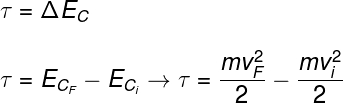ΔE C – Variation of kinetic energy (J)

CF – Final kinetic energy (J)

Ci – Initial kinetic energy (J)

From the relationship shown, known as the work and kinetic energy theorem , we can see that if the kinetic energy of a body remains constant, no work is being done on it, in other words, this body neither receives nor transfers energy from others. bodies.

## Application of the kinetic energy formula

Let’s determine the kinetic energy of a 4 ton (4000 kg) truck moving at a speed of 36 km/h (10 m/s).

### Resolution

First, it is necessary to observe the units, the mass of the body must be written in kilograms and the speed, in meters per second . Next, let’s calculate the kinetic energy: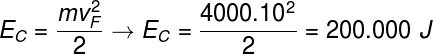## Solved exercises on kinetic energy

Question 1) (Enem 2015) A careful analysis of Usain Bolt’s performance in breaking the 100-meter world record showed that, despite being the last of the runners to react to the shot and start the race, his first 30 meters were the most fastest ever made in a world record, crossing that mark in 3.78 seconds. Until he stood with his body straight, he took 13 strides, showing his power during acceleration, the most important moment of the race. By the end of this course, Bolt had reached a maximum speed of 12 m/s.

Available at: http://esporte.uol.com.br. Accessed on: 5 Aug. 2012

Assuming this runner’s mass is 90 kg, the total work done in the first 13 passes is closer to:

a) 5.4.10 2 J

b) 6.5.10 3 J

c) 8.6.10 2 J

d) 1.3.10 4 J

e) 3.2.10 4 J

Resolution:

alternative b.

Let’s solve the exercise through the theorem of work and kinetic energy, in this way, we must do the following calculation: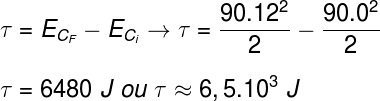Question 2) A body of mass M and kinetic energy E, moves with speed V, reducing the speed of this body to one third of its original value. What should your new kinetic energy E’ be?

a) E/3

b) E/9

c) 9E

d) 3E

e) 16E

Resolution

alternative b.

To solve this exercise, just use the kinetic energy formula, assigning the new speed the value v/3, note: